# 解读经典和隐藏背离的新途径。 第二部分

18 十月 2019, 15:42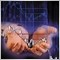0
5 323

### 术语和定义

Alexander Elder 在他的《技术分析中的最强信号》一书中对基于此原理的策略进行了最详细的阐述，该著作里描述了背离和趋势逆转。 读完这本书后，我产生了更多问题。 作者设定了一个强制性条件：指标必须与零轴交叉，然而，在“缺少右肩”一章中，他又说这是背离的可选条件。

A 类背离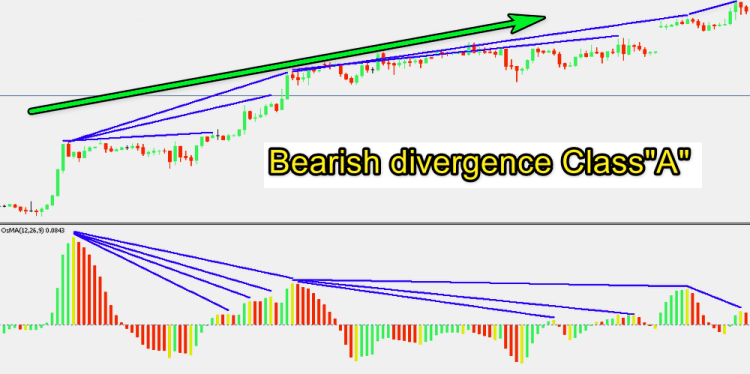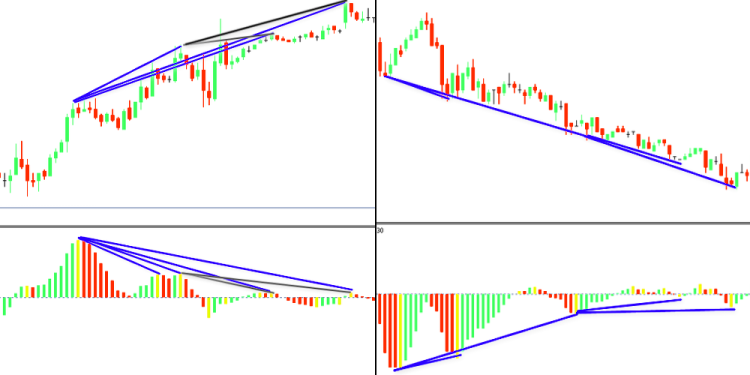B 类背离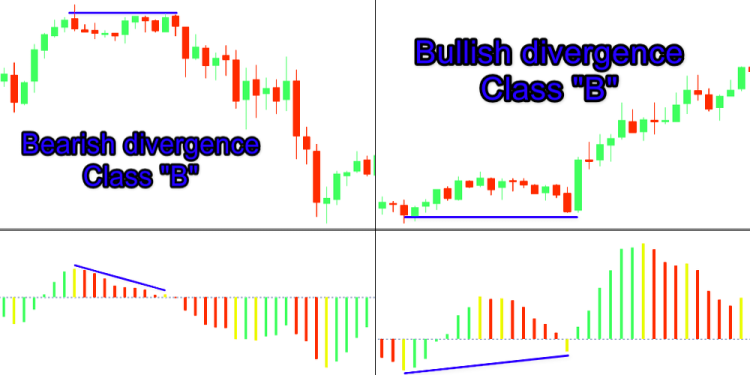C 类背离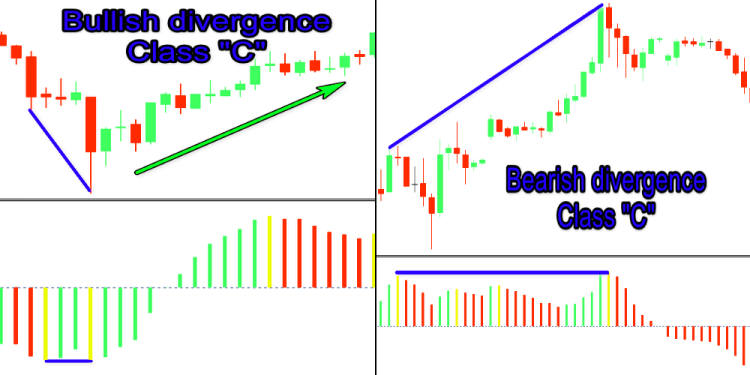### MACD

MACD 指标由 Gerald Appel 创建，用于股票价格分析。 该指标在交易员中非常受欢迎。 受欢迎是出于其有效性经历多年测试的事实，因此，拥有众多的相关资料。 顾名思义，“移动平均收敛/发散”，似乎是专门为此目的而设计的。 指标有两种：线性 MACD 和 MACD 直方图。

• MACD=EMAsn-EMAln
• Signal=EMAmacd
• MACD Histogram:
• MACDn=Signal-MACD

P 是价格，通常等于该期间的收盘价，但也可以使用其他选项（开盘价，最高价，最低价，收盘价，中间价，典型价格，等等）。

• ЕМАs — （短线），周期 12 天（两周）。
• ЕМАl — （长线），周期 26 天（一个月）。
• EМАa — （平滑差价），周期为数值 9。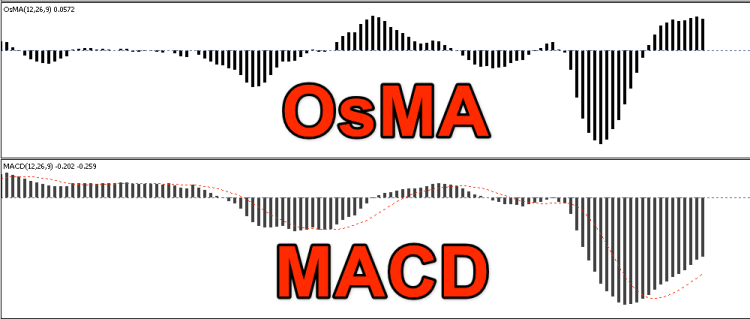MetaTrader 5 MACD 体现为趋势指标，因此，预期经典背离必须准确反映趋势结束。 然而，并非那么简单。 这不仅源于不同的指标解释规则，还源于形态类型的多样性。 在第一部分中，我说过零轴交点是总体趋势结束的明确标志。 这不是真的。 还有一种观点认为，略微的零轴出头能得到更强的逆转信号。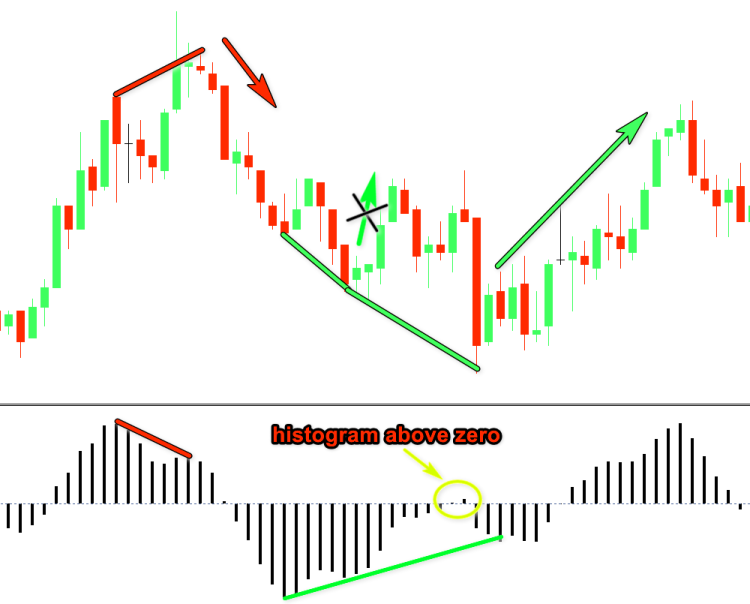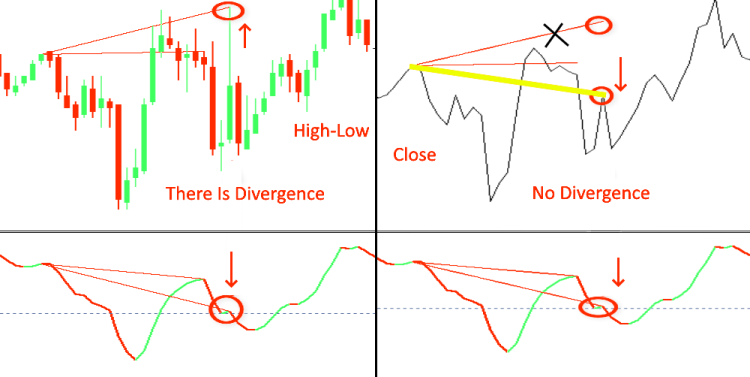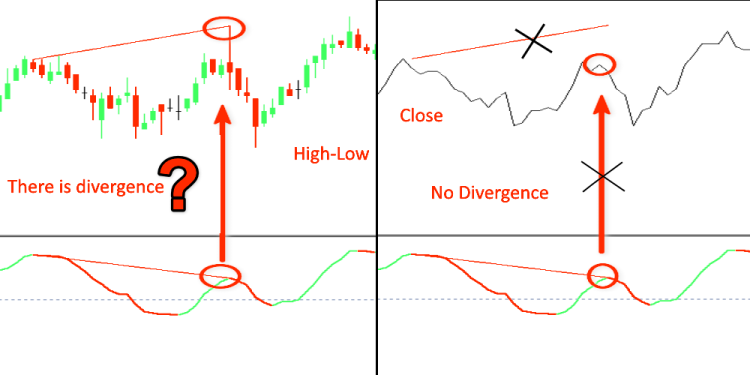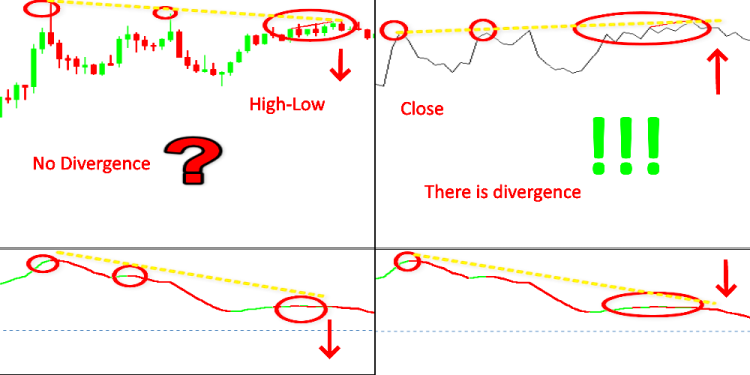`#property indicator_buffers 4`

4 改为 5

`#property indicator_buffers 5`

`#property indicator_type1   DRAW_HISTOGRAM`

`#property indicator_type1   DRAW_COLOR_HISTOGRAM`

`#property indicator_color1  Silver`

`#property indicator_color1  Green,Red,Gold`

```double                   Color_buff[];

```

```   SetIndexBuffer(0,ExtMacdBuffer,INDICATOR_DATA);
SetIndexBuffer(1,Color_buff,INDICATOR_COLOR_INDEX);
SetIndexBuffer(2,ExtSignalBuffer,INDICATOR_DATA);
SetIndexBuffer(3,ExtFastMaBuffer,INDICATOR_CALCULATIONS);
SetIndexBuffer(4,ExtSlowMaBuffer,INDICATOR_CALCULATIONS);

```

```   SetIndexBuffer(1,Color_buff,INDICATOR_COLOR_INDEX);

```

```if(prev_calculated==0)
limit=2;
else limit=prev_calculated-1;

```

```//--- calculate MACD
for(i=limit;i<rates_total && !IsStopped();i++)
{
ExtMacdBuffer[i]=ExtFastMaBuffer[i]-ExtSlowMaBuffer[i];
//--- calculate Color Macd
if(ExtMacdBuffer[i]>ExtMacdBuffer[i-1])
Color_buff[i]=0.0; // set color Green
else
Color_buff[i]=1.0; // set color Red
}
//---

```

```for(i=limit;i<rates_total-1 && !IsStopped();i++)
{
if((ExtMacdBuffer[i-1]<0 && ExtMacdBuffer[i]>ExtMacdBuffer[i-1] && ExtMacdBuffer[i-1]<ExtMacdBuffer[i-2]) ||
(ExtMacdBuffer[i-1]>0 && ExtMacdBuffer[i]<ExtMacdBuffer[i-1] && ExtMacdBuffer[i-1]>ExtMacdBuffer[i-2]))
Color_buff[i-1]=2.0; // set color Gold
}

```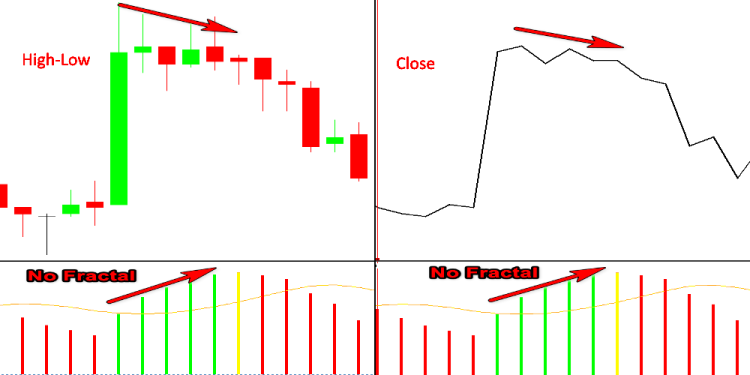• 背离是指振荡器分析的首个波谷比前一个还浅，而相应的波谷价格比前一个波谷价格更深。
• 双重背离是指振荡器形成三个连续波谷，每个波谷都比前一个波谷浅。 而价格形成了三个对应的波谷，每个都比前一个深。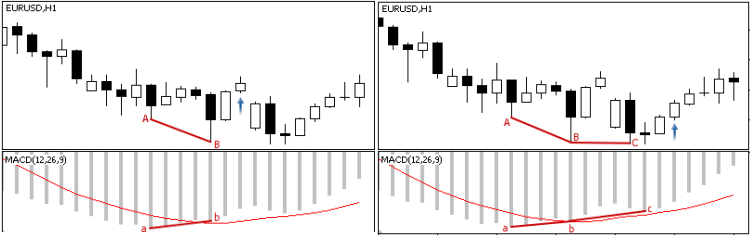图例 11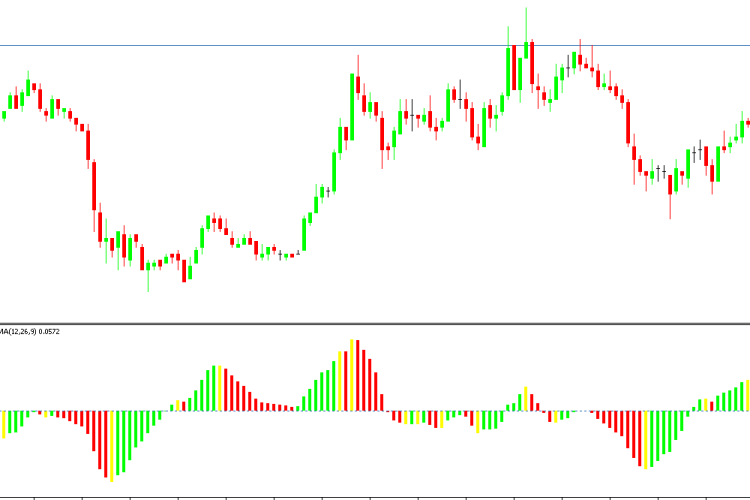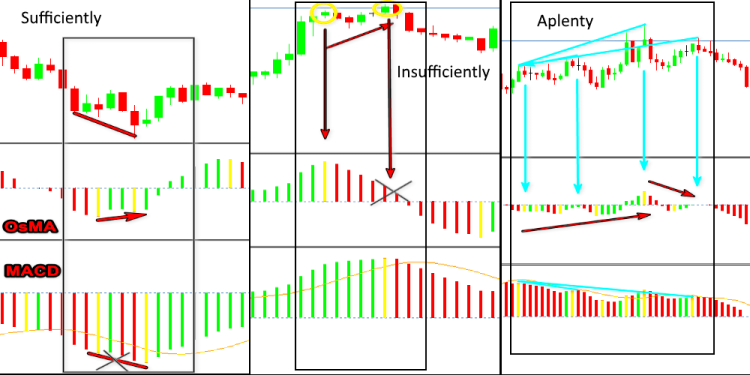### Stochastic（随机振荡）

Stoсhastiс 振荡器是一款技术指标，以百分比的形式衡量价格冲量。 “随机一词是指当期收盘价相对于一段时间内其价格范围的差值。” ，该指标由乔治·莱恩（George Lane）于 2007 年 3 月创建。

• %K Period — 快速随机（实线）。 搜索低点/高点的柱线数量。
• %D Period — 慢速随机指标（虚线）。 应用于 %K.的 SMA 周期
• Slowing — 此参数与 %K 有关。 它设置平滑参数。
• %K=((CLOSEn-LOWn)/(HIGHn-LOWn))*100

• CLOSEn — 前期收盘价：
• LOWn    —  最近 n 个周期的最低价格
• HIGHn   —  最近 n 个周期的最高价格
• %D         — %K.的移动均线 该均线可以使用不同的均化方法：简单均化，指数均化，平滑或线性加权均化。 也可以更改价格序列：高/低或开盘/收盘价。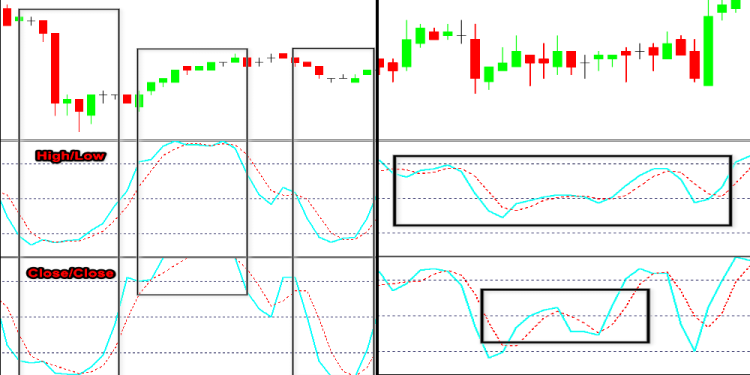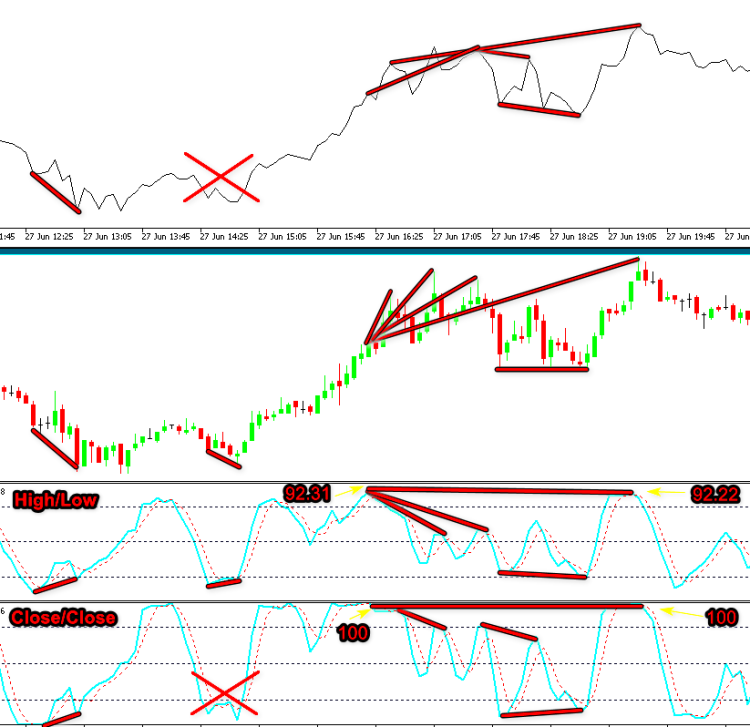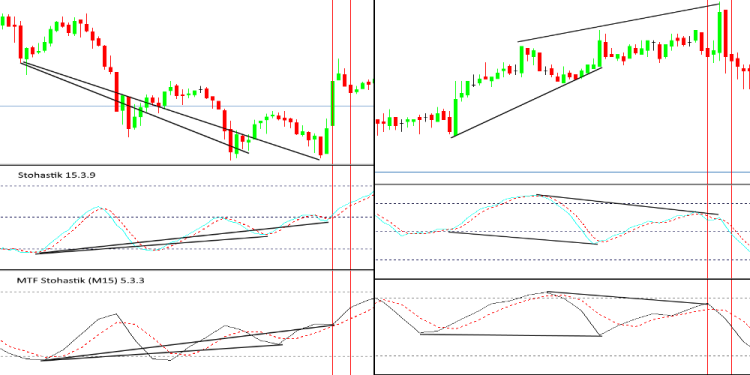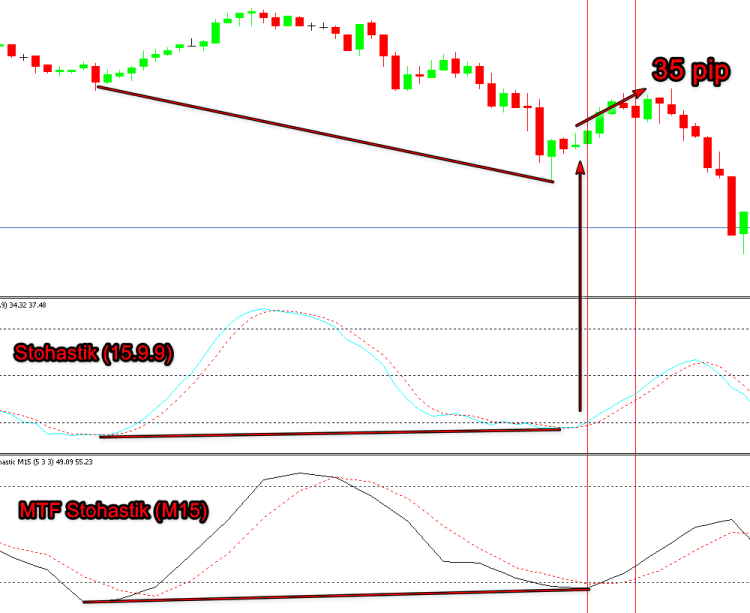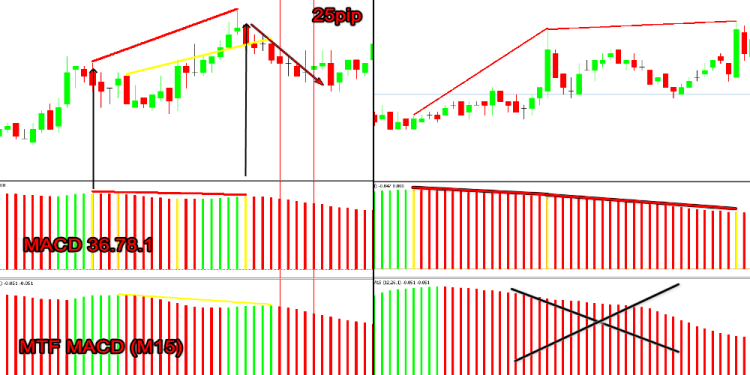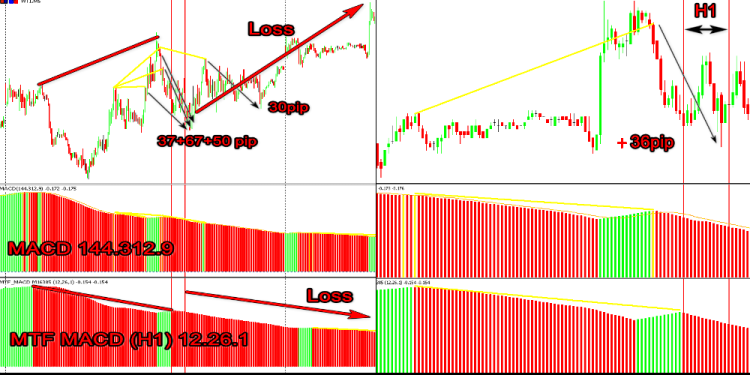1) 从逻辑上讲，我们知道在得到背离（趋同）之前，价格必须达到临界值。 相应地，指标线也需要达到临界水平。 在我们的情况下，指标必须进入超买/超卖区域。 在这种情况下，行情达到新的最高/最低价是可选条件。 在我们的情况下，搜索经典背离时，距当前柱线的第二个点（左侧）必须位于此区域中。 相反，对于隐藏的（逆转）背离，第一点应处于临界水平。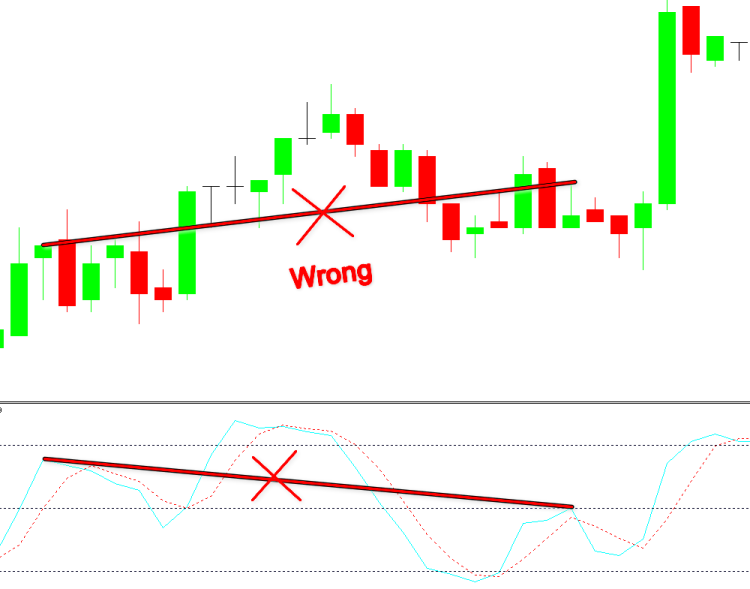2) 搜索信号时（手工或通过算法），搜索最接近极值所需分段应排除图例 20 中情况。 这种序列的最大数目是三个。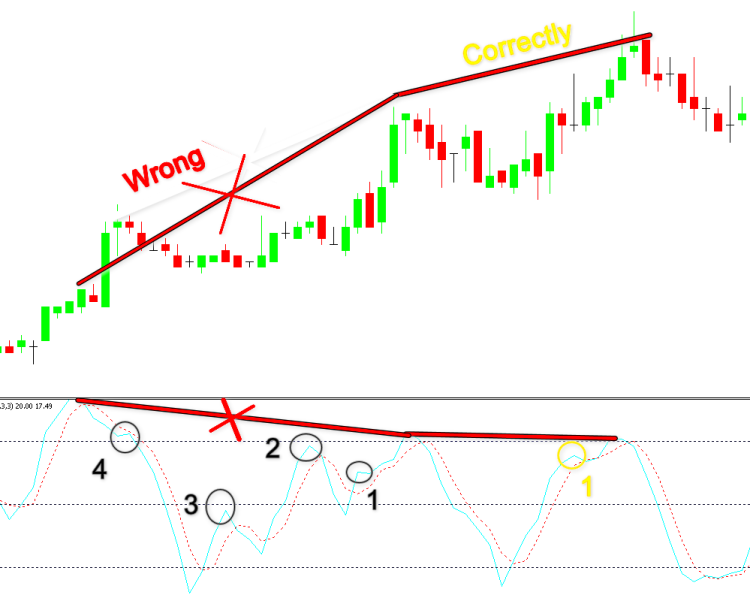### RSI

RSI（相对强度指数）是 J.Welles Wilder 于 1978 年 6 月推出的指标。

RSI=100-100/(1+U/D)

• U 是平均价格在正数范围内的变化值 (CLOSEn>CLOSEn-1 )
• D 是平均价格在负数范围内的变化值 (CLOSEn<CLOSEn-1 )

• RSI=(SMMAn)ofU(SMMAn)ofD
• n - SMMA 计算周期。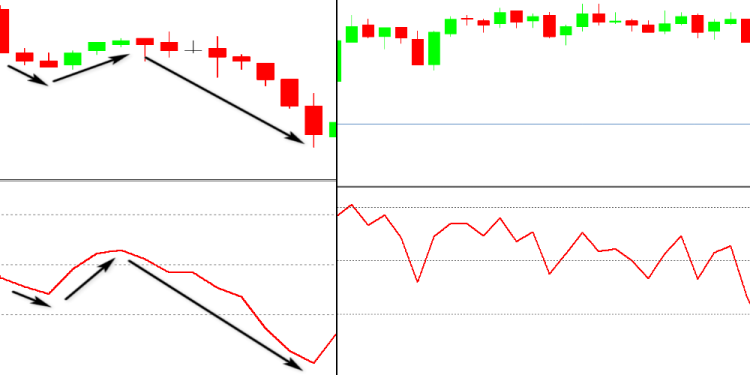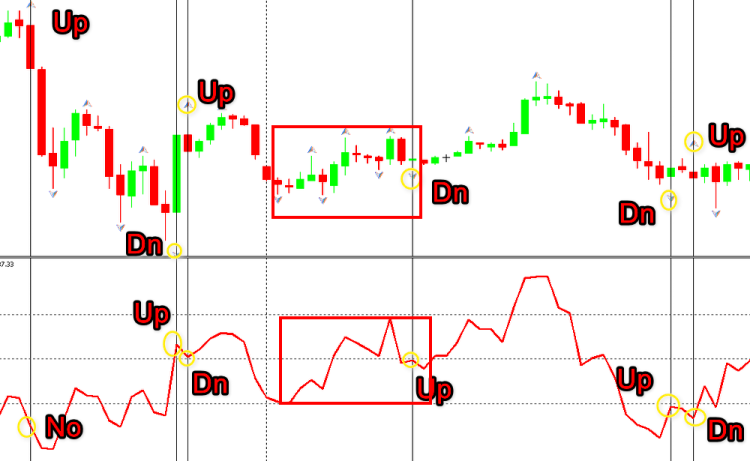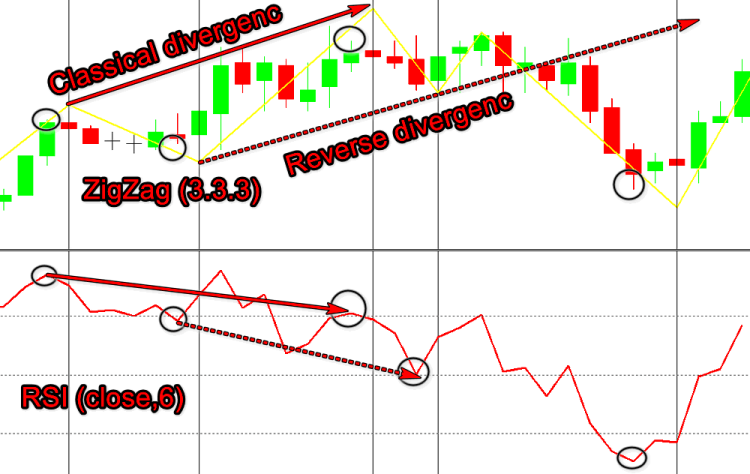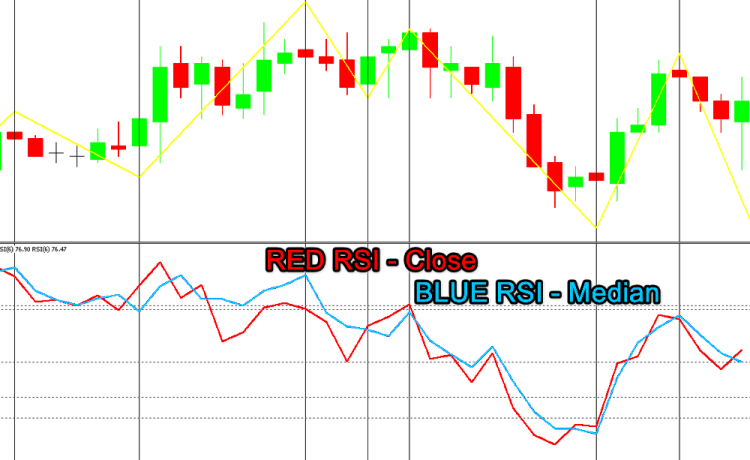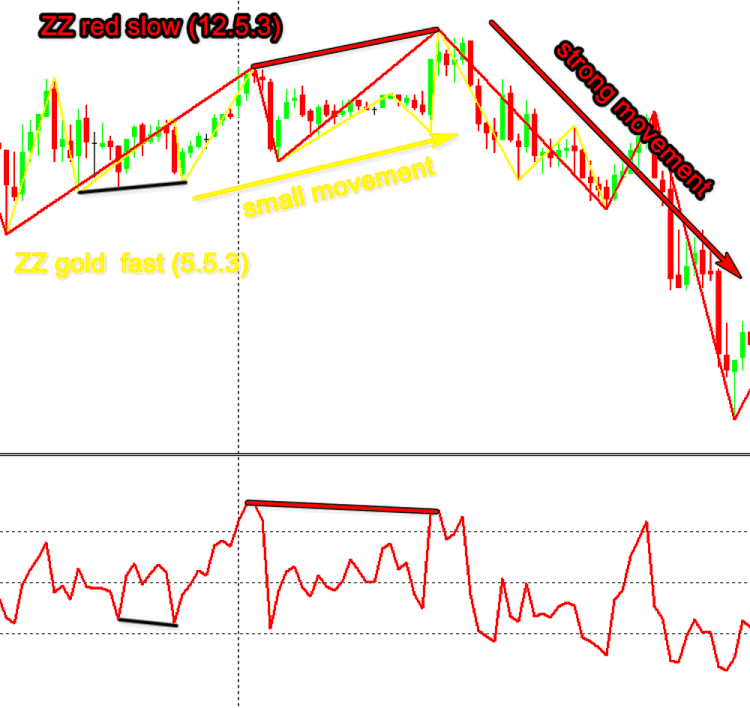### CCI

CCIn=(1/0.015)*((TypicalPrice-SMA)/MedianDeviation)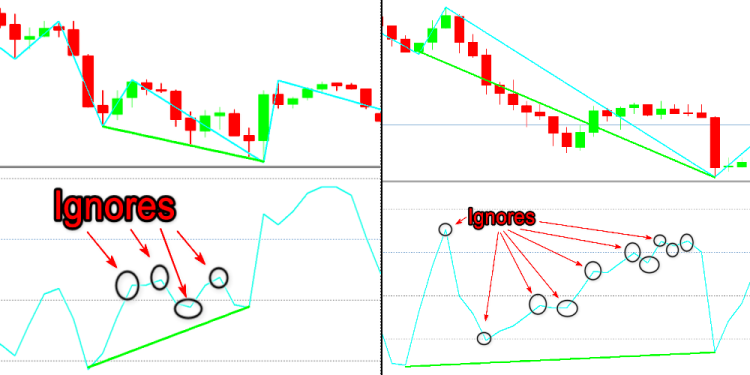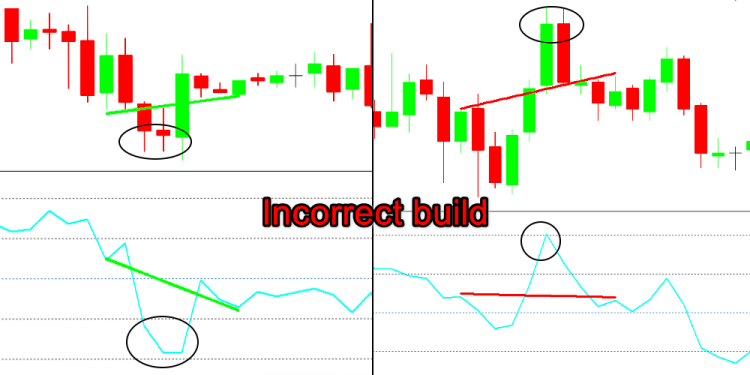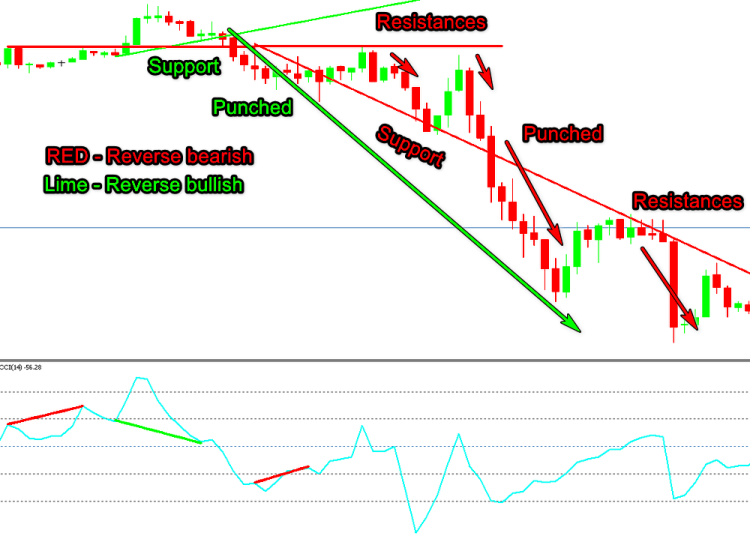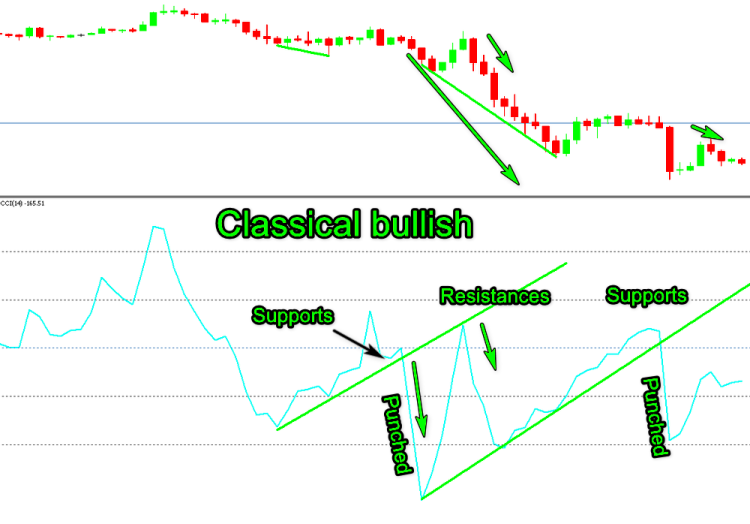### 冲量（Momentum）

Momentum=Pn-Pn-1

• Pt  — 当前价格
• Pt-n — n 个周期前的价格
• RoCtn=(Momentum/Pt-n)*100

• +DI — 正向指数
• -DI  — 负向指数
• MASMA (移动均线)
• n — 均线计算周期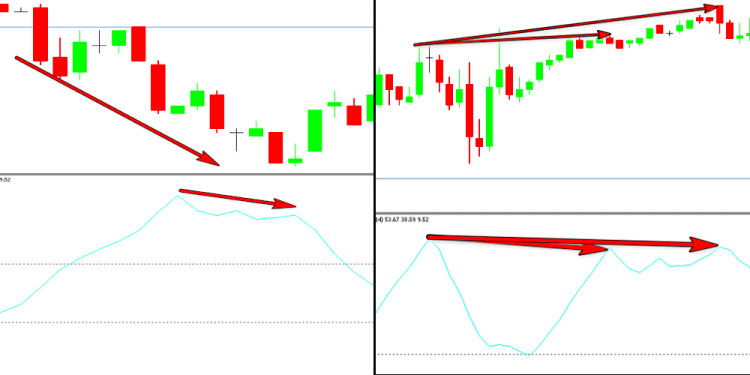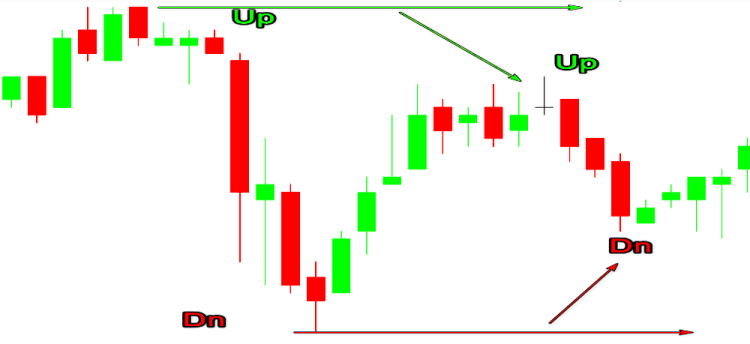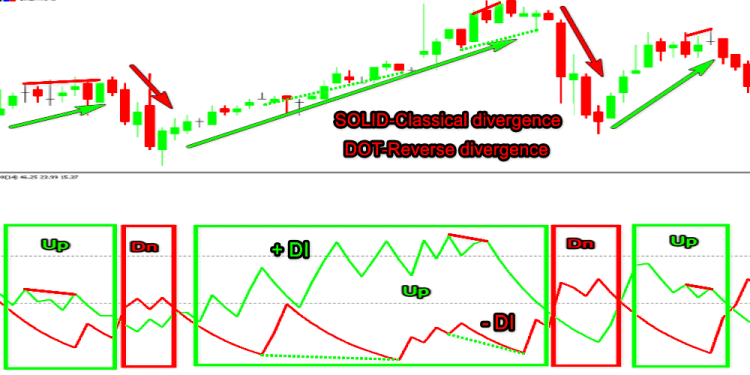WPR(10) 与随机（Stochastic）指标(10.1.1 (最低价/最高价))相同，标尺倒置。 随机指标的范围是 0-100，WPR 的范围是 100-0。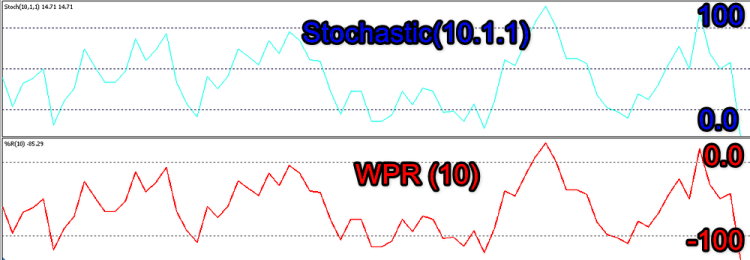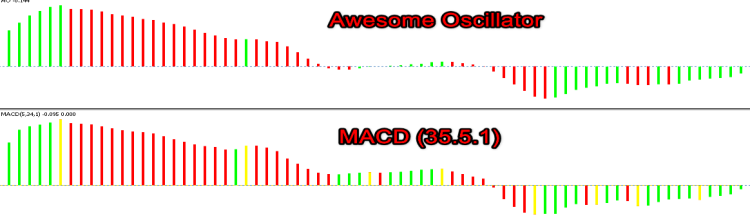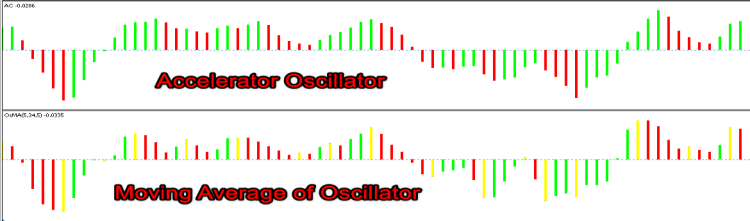TRIX 基于移动平均线，通过选择 MACD 参数可以达到相同的结果。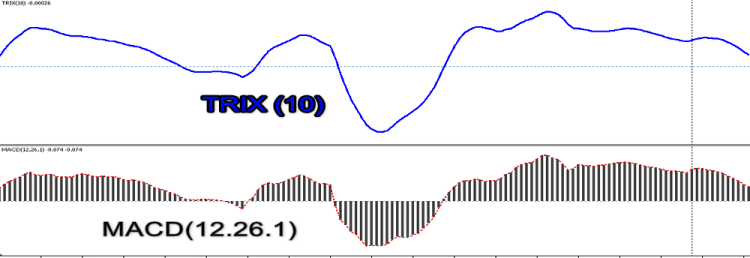### 交易量（Volumes）

MetaTrader 5 终端标准软件包中只有很少的指标在其算法中用到交易量。 其中包括吸筹/派发（Mark Chaikin），资金流指数（由于公式相似，也可称为交易量 RSI），能量潮（Joseph Granville），市场灵活指数（Bill Williams）和交易量。 Alexander Elder 创立的外汇指数也属于这一类，尽管它被包含在“振荡器”部分中。 这种类型的工具很少，因此在分析交易量背离时，我们经常会遇到以下情况：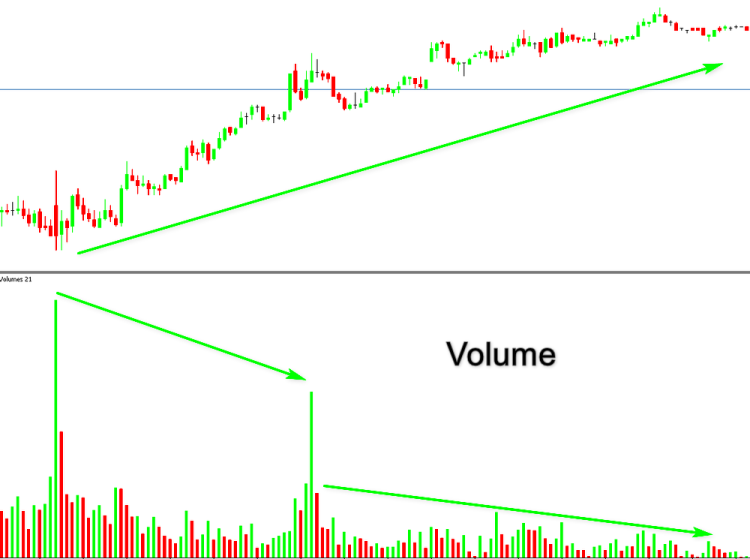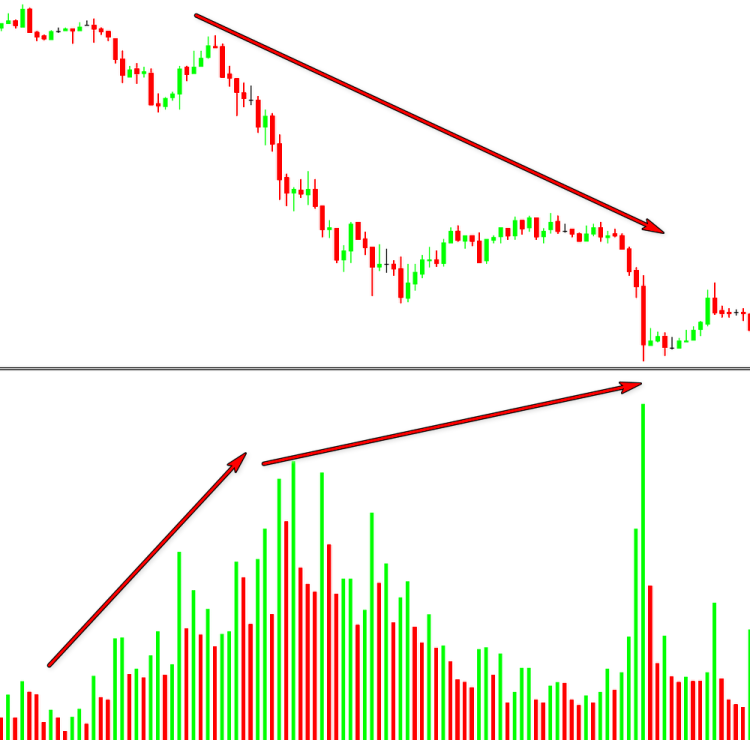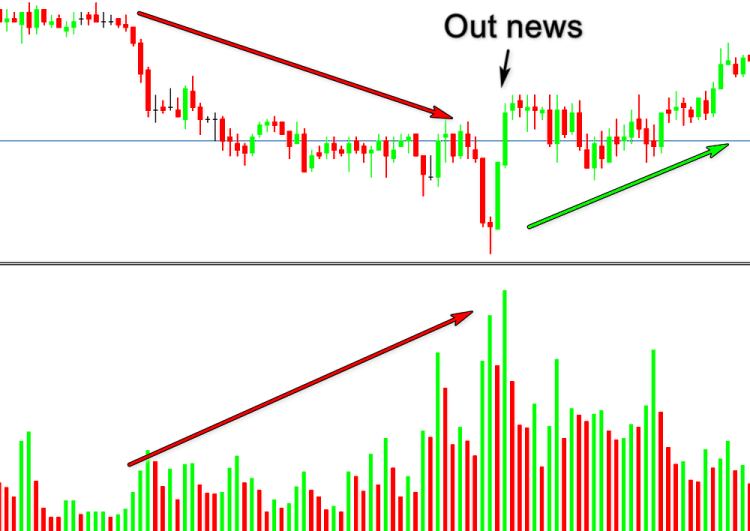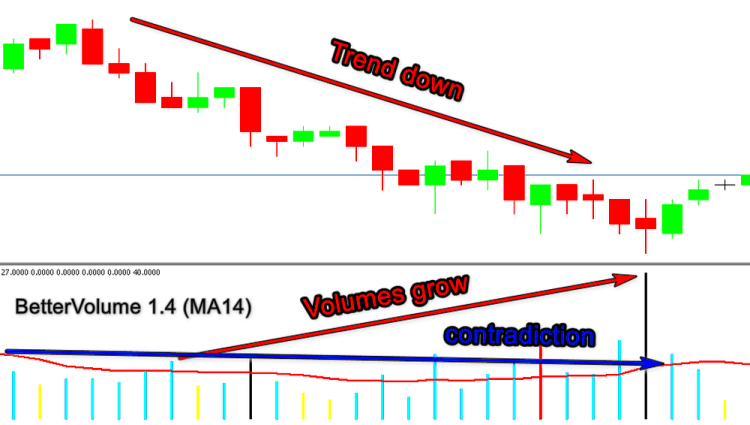```#property indicator_type1   DRAW_COLOR_HISTOGRAM
#property indicator_color1  Green,Red,Blue,Magenta,Gray

```
1. 绿色 - 如果 low[i]>low[i-1] 且 high[i]>high[i-1].
2. 红色     - 如果 low[i]<low[i-1] 且 high[i]<high[i-1].
3. 蓝色   - 外侧向上柱线
4. 粉色 - 外侧向下柱线
5. 灰色 - 以上都不是

```void CalculateVolume(const int nPosition,
const int nRatesCount,
const long &SrcBuffer[],
const double &h[],
const double &l[],
const double &o[],
const double &c[])
{
ExtVolumesBuffer=(double)SrcBuffer;
ExtColorsBuffer=0.0;
//---
for(int i=nPosition;i<nRatesCount && !IsStopped();i++)
{
//--- calculate indicator color
ExtVolumesBuffer[i]=(double)SrcBuffer[i];
ExtColorsBuffer[i]=4.0;
//--- uptrend, UP bar ----
if(h[i]>h[i-1] && l[i]>l[i-1]&& o[i]<c[i])ExtColorsBuffer[i]=0.0;
//--- uptrend, DN bar ----
if(h[i]>h[i-1] && l[i]>l[i-1]&& o[i]>c[i])ExtColorsBuffer[i]=3.0;
//--- downtrend, DN bar ----
if(h[i]<h[i-1] && l[i]<l[i-1]&& o[i]>c[i])ExtColorsBuffer[i]=1.0;
//--- downtrend, UP bar ----
if(h[i]<h[i-1] && l[i]<l[i-1]&& o[i]<c[i])ExtColorsBuffer[i]=2.0;
//--- outside UP bar ----
if(h[i]>h[i-1] && l[i]<l[i-1] && o[i]<c[i])ExtColorsBuffer[i]=2.0;
//--- outside DN bar ----
if(h[i]>h[i-1] && l[i]<l[i-1] && o[i]>c[i])ExtColorsBuffer[i]=3.0;
//--- inside bar ----
if(h[i]<h[i-1] && l[i]>l[i-1])ExtColorsBuffer[i]=4.0;
}
//---
}
//+------------------------------------------------------------------+

```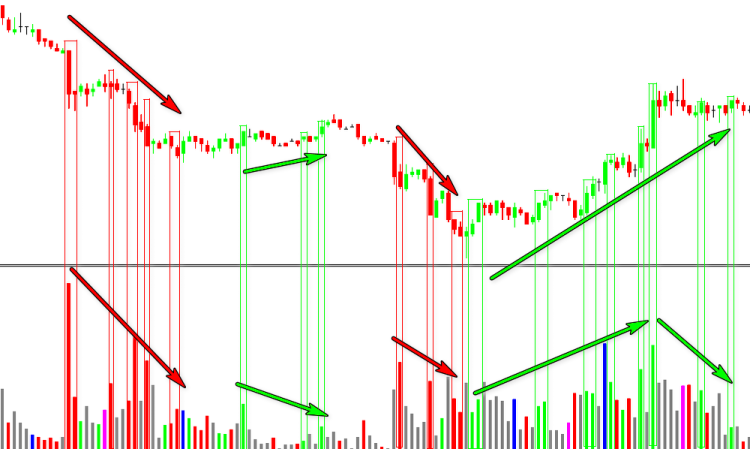MF=价格*交易量

MR=正向MF/负向MF

MFI=100-100/(1+MR)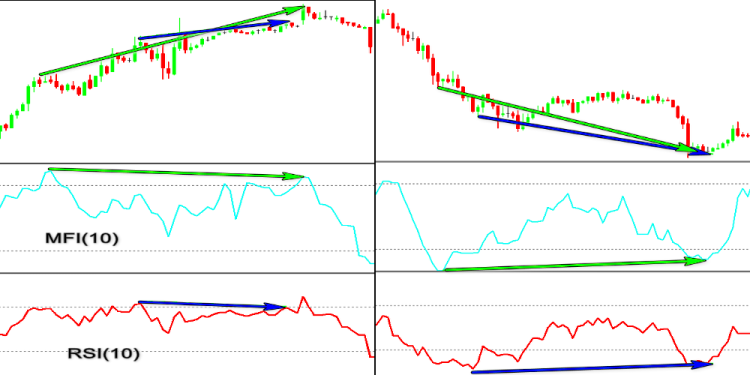(Joseph Granville)

OBVn=OBVn-1-Volumen

OBVn=OBVn-1-Volumen

OBVn=OBVn-1

• OBVn — 当前周期的能量潮指标值；
• OBVn-1 — 前一周期的能量潮指标值；
• Volumen — 当前柱线交易量。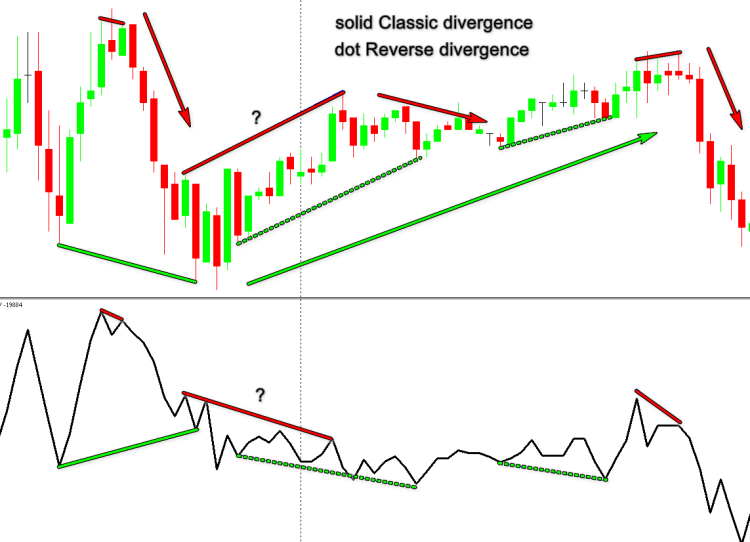(Alexander Elder).

Force Index=Volume*(CLOSEn-CLOSEn-1)

Force Index=Volume*(MAn-MAn-1)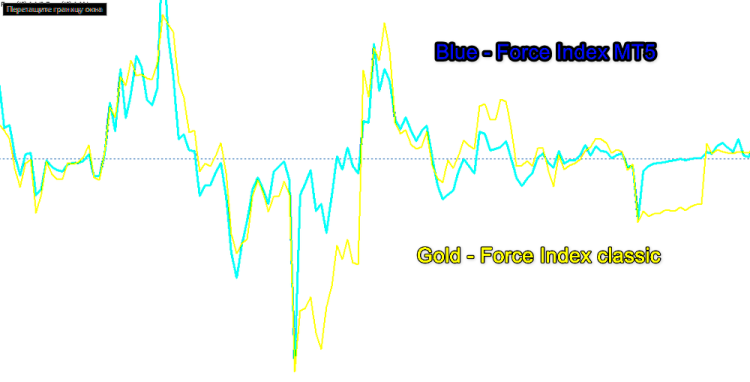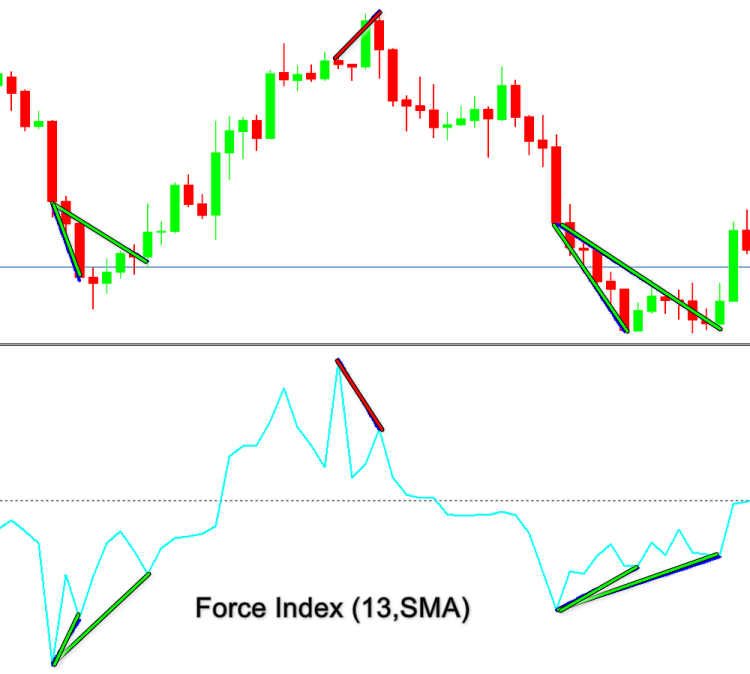### 入场和目标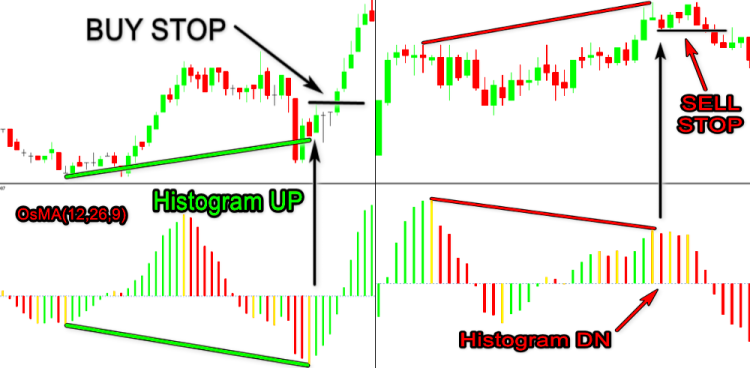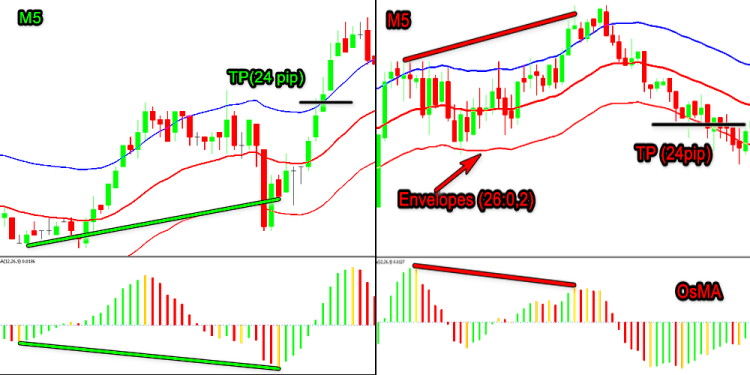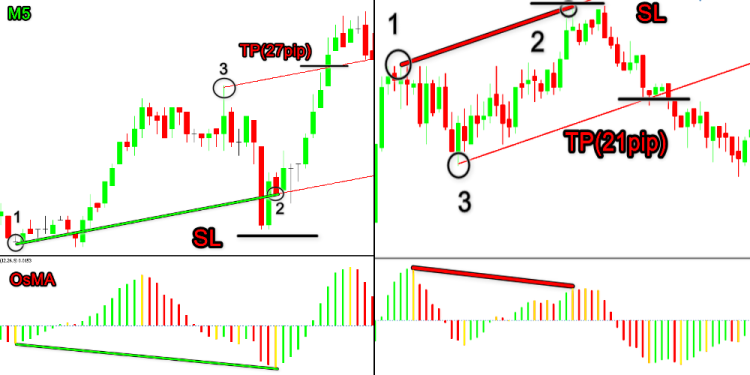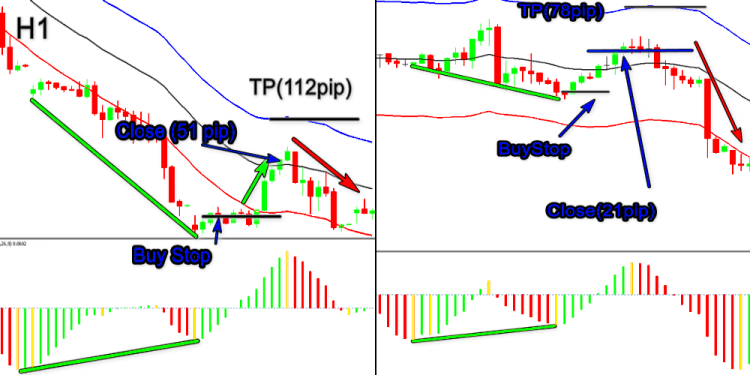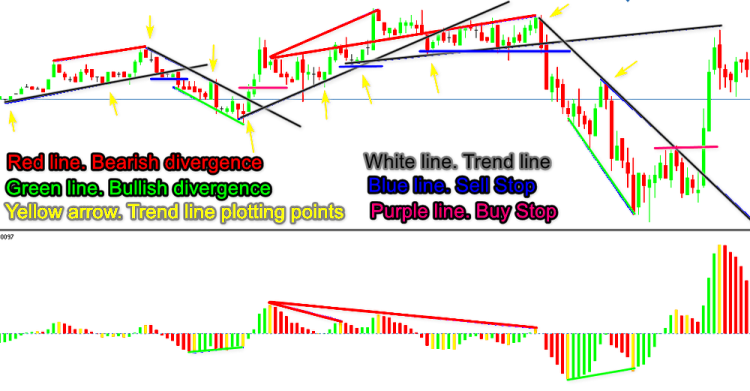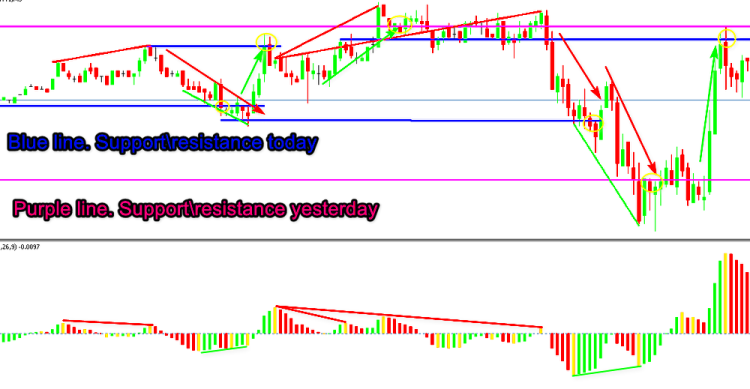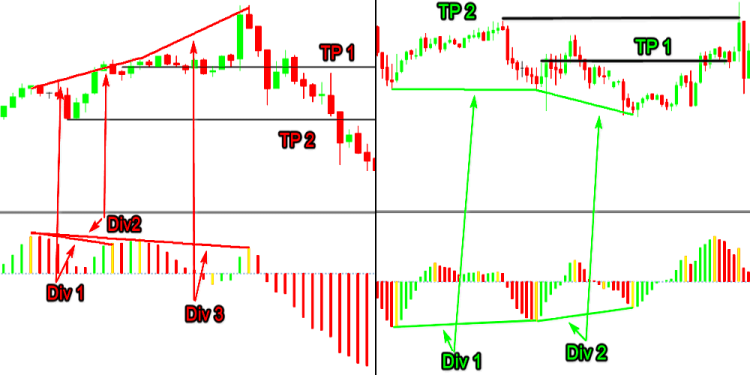### 创建交易工具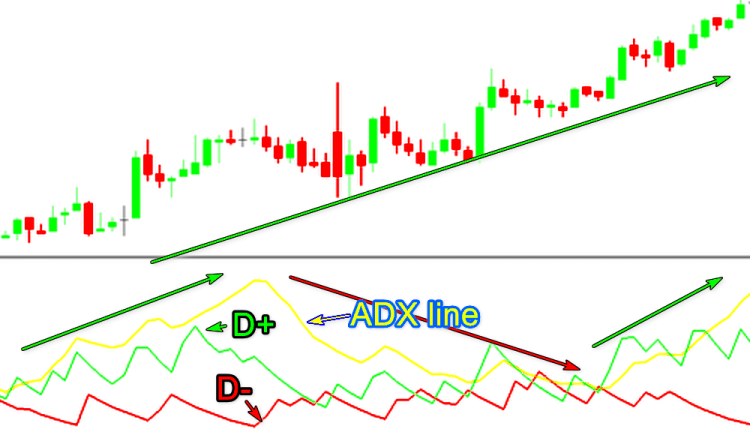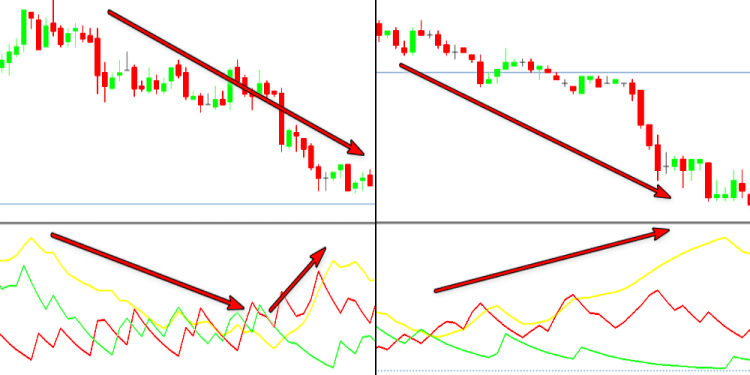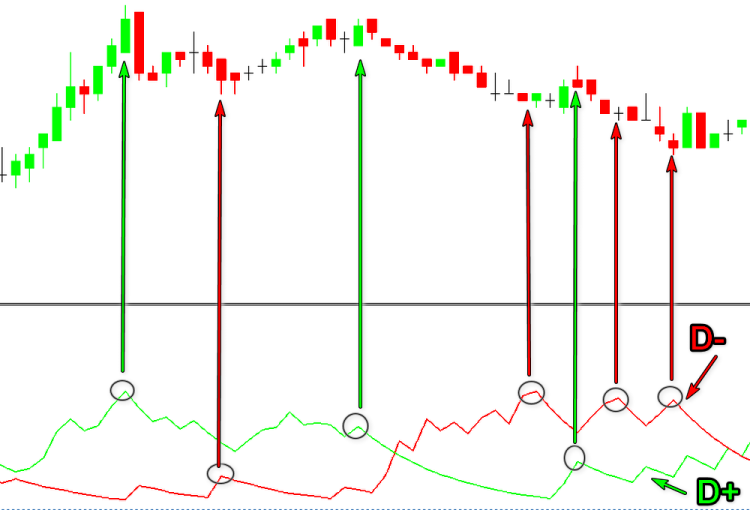`         dTmp=100.0*MathAbs((ExtPDIBuffer[i]-ExtNDIBuffer[i])/dTmp);`

MathAbs 函数。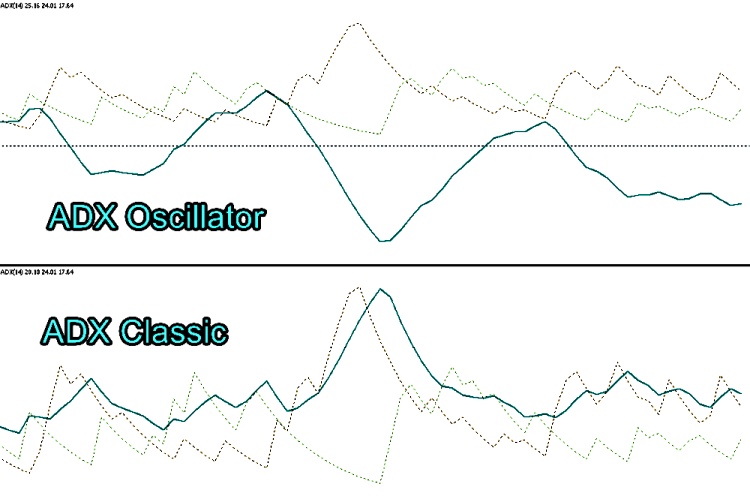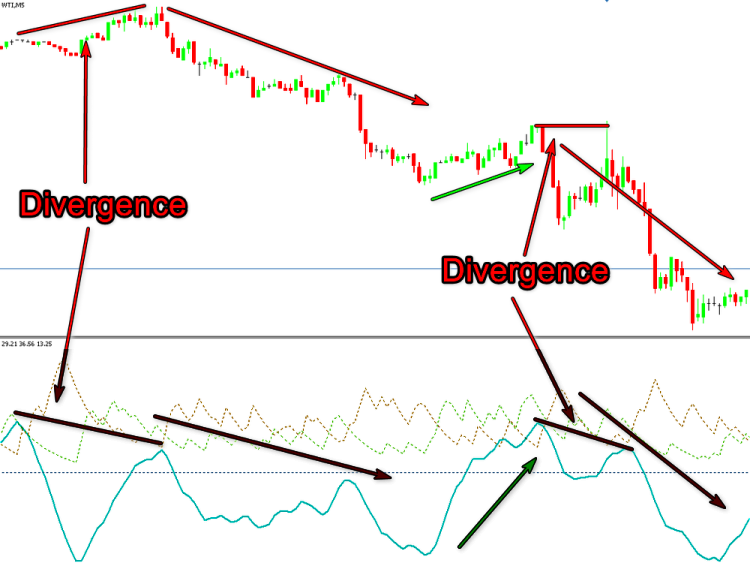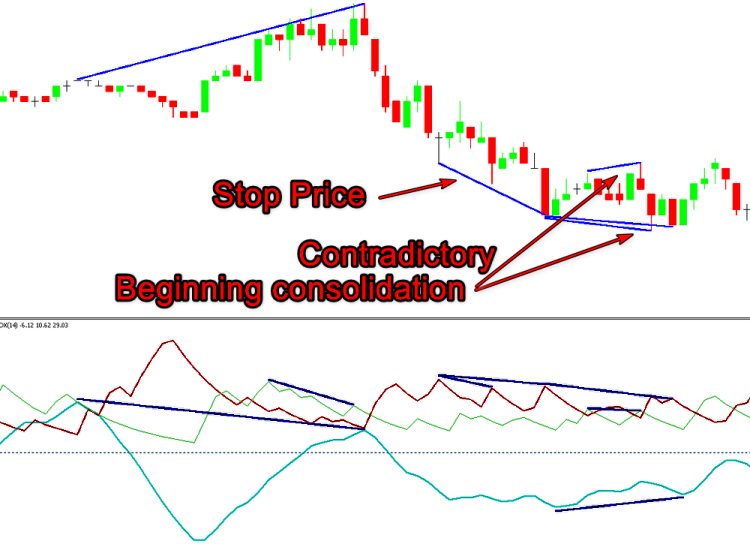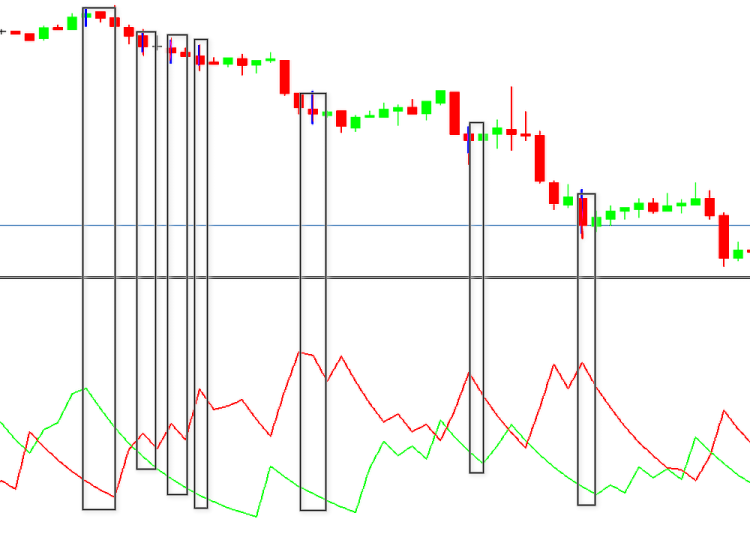```#property indicator_separate_window
#property indicator_buffers 8
#property indicator_plots   3
#property indicator_type1   DRAW_LINE
#property indicator_color1  LightSeaGreen
#property indicator_style1  STYLE_SOLID
#property indicator_width1  1
#property indicator_type2   DRAW_LINE
#property indicator_color2  Green
#property indicator_style2  STYLE_DOT
#property indicator_width2  1
#property indicator_type3   DRAW_LINE
#property indicator_color3  Red
#property indicator_style3  STYLE_DOT
#property indicator_width3  1
#property indicator_label2  "+DI"
#property indicator_label3  "-DI"
//--- input parameters
input int extremum=100; // Extremum (<1 Div= OFF)
input int Gep=4; // Point to Gep
input int Bars_Calculated=300;
//--- global variables
input bool   allLine=false;// All Line
double PDI,NDI,pDI,nDI;
string short_name="";
int    wid=-1;
//---- buffers
double    ExtPDIBuffer[];
double    ExtNDIBuffer[];
double    ExtPDBuffer[];
double    ExtNDBuffer[];
double    ExtTmpBuffer[];
double    ExtUpBuffer[];
double    ExtDnBuffer[];

```

```input int extremum=100; // Extremum (<1 Div= OFF)

```

`input bool   allLine=false;// All Line`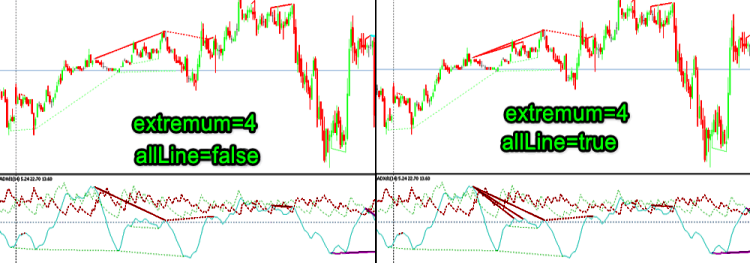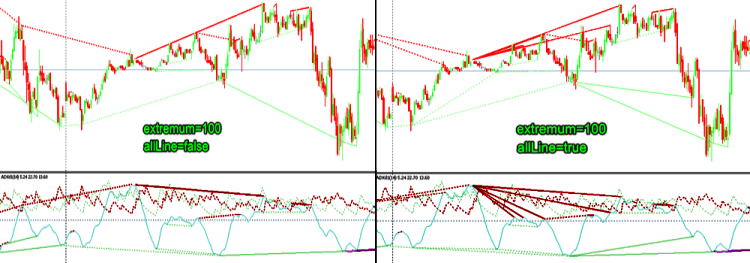`input int Gep=4; // Point to Gep`

```//+------------------------------------------------------------------+
void OnDeinit(const int reason)
{
ObjectsDeleteAll(0,short_name);
ChartRedraw(0);
}
//+------------------------------------------------------------------+

```

```   if(prev_calculated>1) start=prev_calculated-1;
else
{
OnDeinit(9);
start=1;
ExtPDIBuffer=0.0;
ExtNDIBuffer=0.0;
}

```

```//+------------------------------------------------------------------+
//|           Fast divergence calculation                            |
//+------------------------------------------------------------------+
int bar=start;
if(prev_calculated<2) bar=start+2;
if(Bars_Calculated>0)bar=fmax(rates_total-Bars_Calculated,start);
//---
for(int i=bar;i<rates_total-1 && !IsStopped();i++)
{
ExtUpBuffer[i-1]=EMPTY_VALUE;
ExtDnBuffer[i-1]=EMPTY_VALUE;
//---
color clrUp=clrLime;
color clrDn=clrDarkViolet;
//---
if((ExtPDIBuffer[i-1]<ExtPDIBuffer[i-2] && ExtNDIBuffer[i-1]<ExtNDIBuffer[i-2]) ||
(ExtPDIBuffer[i-1]>ExtPDIBuffer[i-2] && ExtNDIBuffer[i-1]>ExtNDIBuffer[i-2]))
{
if(ExtPDIBuffer[i-1]>ExtNDIBuffer[i-1])
{
ExtUpBuffer[i-1]=high[i-1];
else
{
ExtUpBuffer[i-1]=EMPTY_VALUE;
ExtDnBuffer[i-1]=low[i-1];
clrDn=clrGold;
}

//---
if(MathAbs(ExtUpBuffer[i-1]-ExtUpBuffer[i-2])<Gep*_Point)
ExtUpBuffer[i-1]=ExtUpBuffer[i-2];
if(MathAbs(ExtDnBuffer[i-1]-ExtDnBuffer[i-2])<Gep*_Point)
ExtDnBuffer[i-1]=ExtDnBuffer[i-2];
//---
}
if(ExtPDIBuffer[i-1]<ExtNDIBuffer[i-1])
{
ExtDnBuffer[i-1]=low[i-1];
else
{
ExtDnBuffer[i-1]=EMPTY_VALUE;
ExtUpBuffer[i-1]=high[i-1];
clrUp=clrBlue;
}

//---
if(MathAbs(ExtDnBuffer[i-1]-ExtDnBuffer[i-2])<Gep*_Point)
ExtDnBuffer[i-1]=ExtDnBuffer[i-2];
if(MathAbs(ExtUpBuffer[i-1]-ExtUpBuffer[i-2])<Gep*_Point)
ExtUpBuffer[i-1]=ExtUpBuffer[i-2];
//---
}
}
//---
if(ExtUpBuffer[i-1]==EMPTY_VALUE)
ExtUpBuffer[i-1]=ExtUpBuffer[i-2];
if(ExtDnBuffer[i-1]==EMPTY_VALUE)
ExtDnBuffer[i-1]=ExtDnBuffer[i-2];
//---
if(ExtUpBuffer[i-1]!=EMPTY_VALUE)
{
ArrowCreate(short_name+(string)time[i]+(string)Lime,time[i],ExtUpBuffer[i-1],ANCHOR_BOTTOM,clrUp);
if(ExtUpBuffer[i-1]!=ExtUpBuffer[i-2])
ArrowCreate(short_name+(string)time[i-1]+(string)Lime,time[i-1],ExtUpBuffer[i-1],ANCHOR_BOTTOM,clrUp);
}
else
ArrowCreate(short_name+(string)time[i]+(string)Lime,time[i],ExtUpBuffer[i-2],ANCHOR_BOTTOM,clrUp);
//---
if(ExtDnBuffer[i-1]!=EMPTY_VALUE)
{
ArrowCreate(short_name+(string)time[i]+(string)Red,time[i],ExtDnBuffer[i-1],ANCHOR_TOP,clrDn);
if(ExtDnBuffer[i-1]!=ExtDnBuffer[i-2])
ArrowCreate(short_name+(string)time[i-1]+(string)Red,time[i-1],ExtDnBuffer[i-1],ANCHOR_TOP,clrDn);
}
else
ArrowCreate(short_name+(string)time[i]+(string)Red,time[i],ExtDnBuffer[i-2],ANCHOR_TOP,clrDn);
}

```

```//+-------------------- Finding a discrepancy ----------------------+
bar=start;
if(bar>rates_total-2)bar=rates_total-2;
if(Bars_Calculated>0)bar=fmax(rates_total-Bars_Calculated,bar);
for(int i=bar;i<rates_total-2 && !IsStopped() && extremum>0;i++)
{
}

```

```//+----------- Detecting UP divergences ------------------------------+
void UP(int l,const double &buf[],const double &high[],const datetime &time[])
{
if(Extremum(buf[l+1],buf[l],buf[l-1])<0)
{
int i=l;
for(int j=0;j<extremum;j++)
{
int counted=LastPeak(i,buf);
if(counted!=-1)
{
if(buf[l]<buf[counted] && high[l]>high[counted])
{
double k=fabs(buf[l]-buf[counted])/(l-counted);
int z=1;
bool yes=true;
for(i=l-1;i>counted;z++,i--)
if(buf[i]>buf[l]+k*z){yes=false;break;}
if(yes)
{
DrawPriceTrendLine(time[l],time[counted],high[l],high[counted],Red,STYLE_SOLID);
DrawIndicatorTrendLine(time[l],time[counted],buf[l],buf[counted],Red,STYLE_SOLID);
break;
}
}

if(buf[l]>buf[counted] && high[l]<high[counted])
{
double k=fabs(buf[l]-buf[counted])/(l-counted);
int z=1;
bool yes=true;
for(i=l-1;i>counted;z++,i--)
if(buf[i]>buf[l]-k*z){yes=false;break;}
if(yes)
{
DrawPriceTrendLine(time[l],time[counted],high[l],high[counted],Red,STYLE_DOT);
DrawIndicatorTrendLine(time[l],time[counted],buf[l],buf[counted],Red,STYLE_DOT);
break;
}
}
}
i=counted;
}
//---
}
}

```

```//+----------- Detecting DN divergences ------------------------------+
void DN(int l,const double &buf[],const double &low[],const datetime &time[])
{
if(Extremum(buf[l+1],buf[l],buf[l-1])>0)
{
int i=l;
//---
for(int j=0;j<extremum;j++)
{
int counted=LastTrough(i,buf);
if(counted!=-1)
{
if(buf[l]>buf[counted] && low[l]<low[counted])
{
double k=fabs(buf[l]-buf[counted])/(l-counted);
int z=1;
bool yes=true;
for(i=l-1;i>counted;z++,i--)
if(buf[i]<buf[l]-k*z){yes=false;break;}
if(yes)
{
DrawPriceTrendLine(time[l],time[counted],low[l],low[counted],Green,STYLE_SOLID);
DrawIndicatorTrendLine(time[l],time[counted],buf[l],buf[counted],Green,STYLE_SOLID);
break;
}
}
if(buf[l]<buf[counted] && low[l]>low[counted])
{
double k=fabs(buf[l]-buf[counted])/(l-counted);
int z=1;
bool yes=true;
for(i=l-1;i>counted;z++,i--)
if(buf[i]<buf[l]+k*z){yes=false;break;}
if(yes)
{
DrawPriceTrendLine(time[l],time[counted],low[l],low[counted],Green,STYLE_DOT);
DrawIndicatorTrendLine(time[l],time[counted],buf[l],buf[counted],Green,STYLE_DOT);
break;
}
}
}
i=counted;
}
//---
}
}

```

```//+-- Search for extrema --------------------------------------------+
int Extremum(double a,double b,double c)
{
if((a-b)*(b-c)<=0)
{
if(c>b) return(1); //DN extremum
if(c<b) return(-1);//UP extremum
}
return(0);
}

```

```//+----- Search for the second UP extremum --------------------------+
int LastPeak(int l,const double &buf[])
{
for(int i=l-2; i>4; i--)
{
int ext=Extremum(buf[i+1],buf[i],buf[i-1]);
if(ext < 0)return (i);
}
return (-1);
}
//+----- Search for the second DN extremum --------------------------+
int LastTrough(int l,const double &buf[])
{
for(int i=l-2; i>4; i--)
{
int ext=Extremum(buf[i+1],buf[i],buf[i-1]);
if(ext > 0)return (i);

}
return (-1);
}

```

```//+-------------------- Creates an arrow -----------------------------+
bool ArrowCreate(string                  name,
datetime                time,
double                  price,
ENUM_ARROW_ANCHOR       anchor,
color                   clr)
{
//--- reset the error value
ResetLastError();
//--- create an arrow
if(!ObjectCreate(0,name,OBJ_ARROW,0,time,price))
{
Print(__FUNCTION__,
": failed to create an arrow! Error code = ",GetLastError());
return(false);
}
ObjectSetInteger(0,name,OBJPROP_ARROWCODE,167);
//--- set the binding type
ObjectSetInteger(0,name,OBJPROP_ANCHOR,anchor);
ObjectSetInteger(0,name,OBJPROP_COLOR,clr);
ObjectSetInteger(0,name,OBJPROP_STYLE,STYLE_SOLID);
ObjectSetInteger(0,name,OBJPROP_WIDTH,1);
ObjectSetInteger(0,name,OBJPROP_BACK,false);
ObjectSetInteger(0,name,OBJPROP_SELECTABLE,false);
ObjectSetInteger(0,name,OBJPROP_SELECTED,false);
ObjectSetInteger(0,name,OBJPROP_HIDDEN,true);
//--- successful implementation
return(true);
}
//+------------------------------------------------------------------+

```

```//+------ Creating objects on the price chart -----------------------+
void DrawPriceTrendLine(datetime T_0,datetime T_1,double P_0,double P_1,color color_0,int style)
{
string name_0=short_name+"Line_Sn"+ColorToString(color_0);
string name_1="";
if(allLine)
name_1=short_name+DoubleToString(T_0,0);
else
name_1=short_name+DoubleToString(T_1,0);
//---
ObjectDelete(0,name_1);
drawLineS(name_1,T_0,T_1,P_0,P_1,color_0,style,0,true,false,0);
//+-----------+
if(style==STYLE_DOT)
{
ObjectDelete(0,name_0);
drawLineS(name_0,T_1,T_0,P_1,P_0,clrAqua,0,3,true,true,0);
}
}

```

```//+------ Creating objects in the indicator window ------------------+
void DrawIndicatorTrendLine(datetime T_0,datetime T_1,double P_0,double P_1,color color_0,int style)
{

int window=wid;
string name_0=short_name+"Line_Pn"+ColorToString(color_0);
string name_1="";
if(allLine)
name_1=short_name+DoubleToString(T_0+wid,0);
else
name_1=short_name+DoubleToString(T_1+wid,0);
//---
ObjectDelete(0,name_1);
drawLineS(name_1,T_0,T_1,P_0,P_1,color_0,style,0,false,false,window);
//---
if(style==STYLE_SOLID)
{
ObjectDelete(0,name_0);
drawLineS(name_0,T_1,T_0,P_1,P_0,clrMagenta,style,2,true,true,window);
}
}

```

```//+------------------------------------------------------------------+
void drawLineS(string name,datetime T_0,datetime T_1,double P_0,double P_1,color clr,
int style,int width,bool back,bool ray,int window)
{
ObjectCreate(0,name,OBJ_TREND,window,T_0,P_0,T_1,P_1,0,0);
ObjectSetInteger(0,name,OBJPROP_RAY_RIGHT,ray);
ObjectSetInteger(0,name,OBJPROP_COLOR,clr);
ObjectSetInteger(0,name,OBJPROP_STYLE,style);
ObjectSetInteger(0,name,OBJPROP_WIDTH,width);
ObjectSetInteger(0,name,OBJPROP_SELECTABLE,false);
ObjectSetInteger(0,name,OBJPROP_BACK,back);
}

```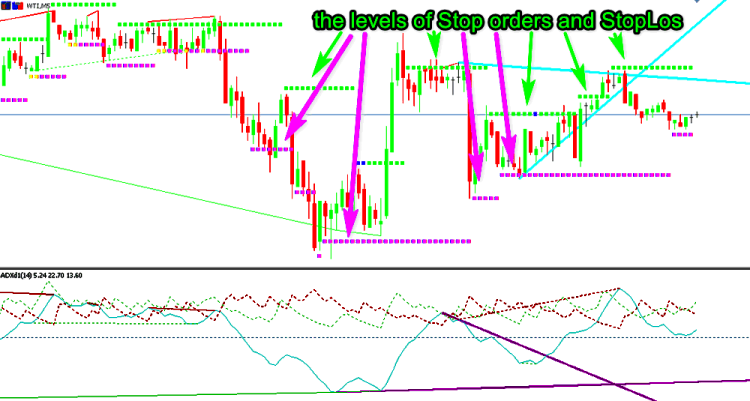EURUSD Н1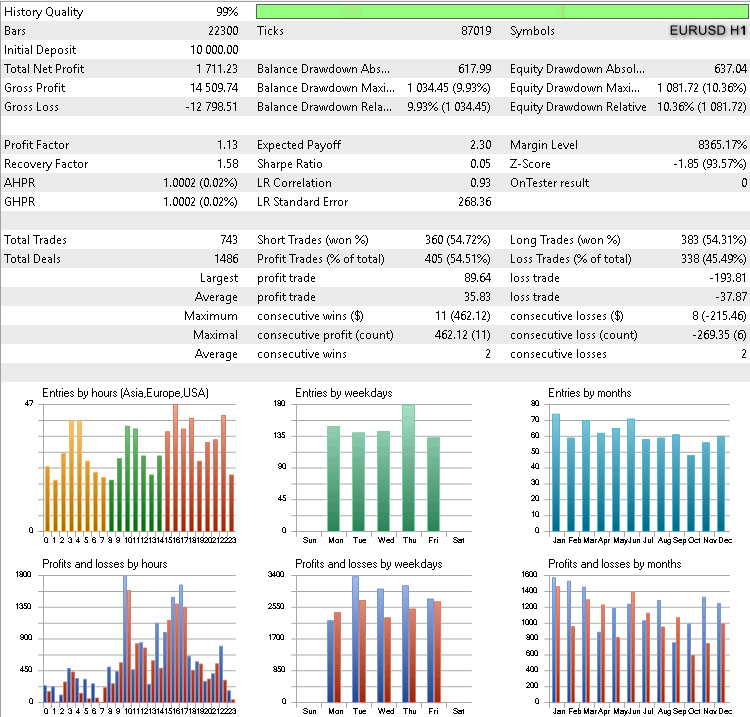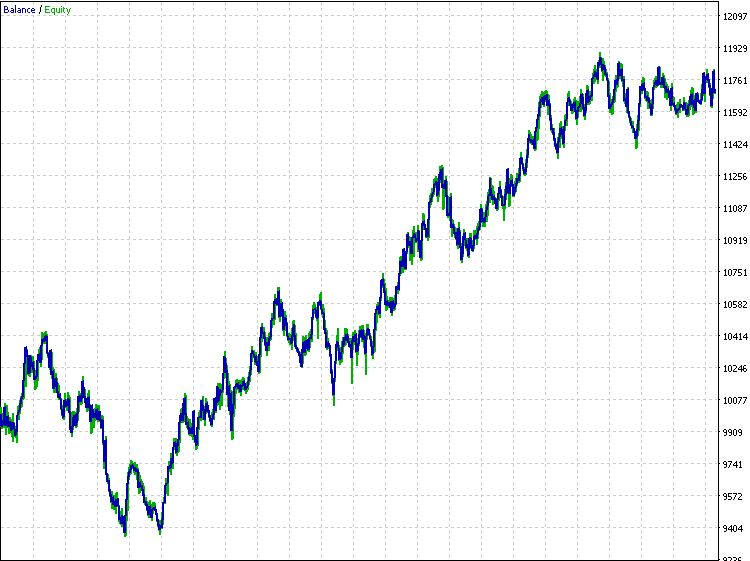EURUSD Н4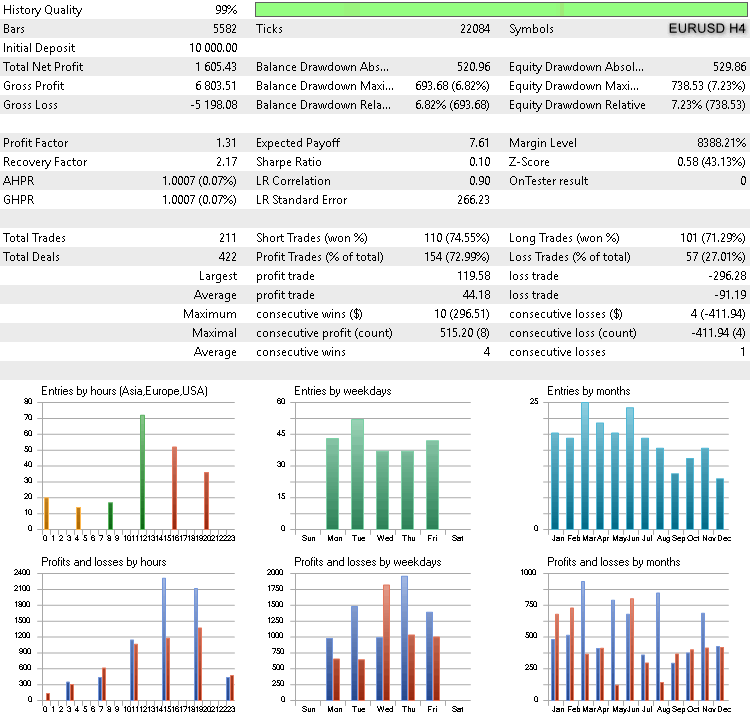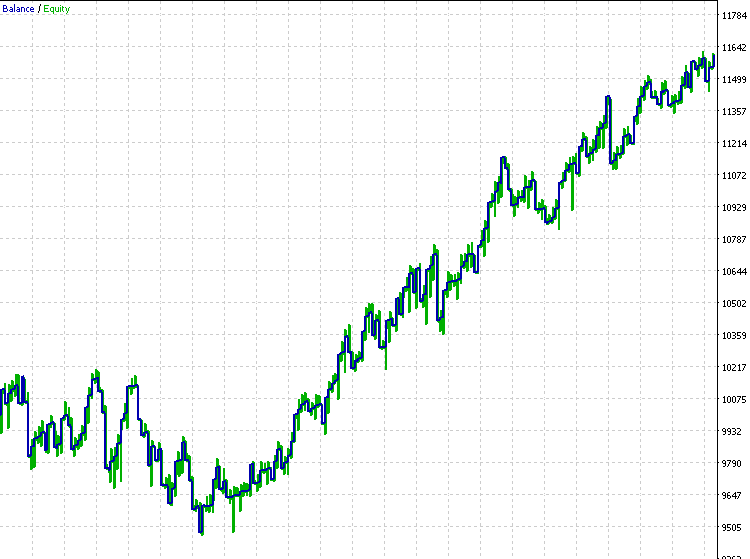GBPUSD H1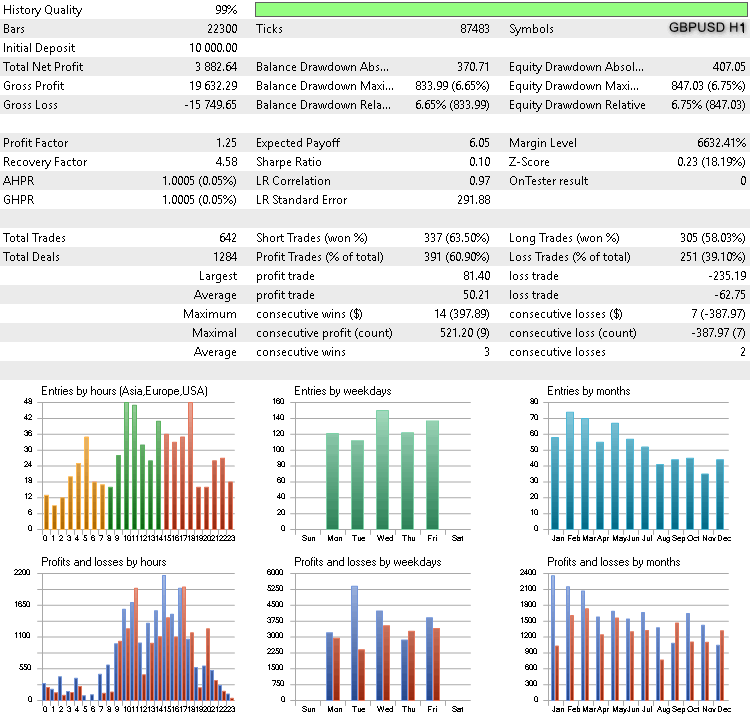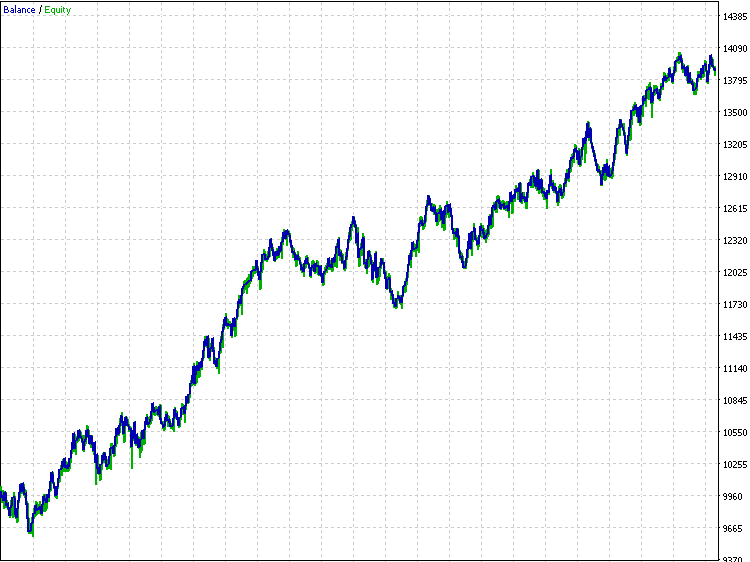GBPUSD H4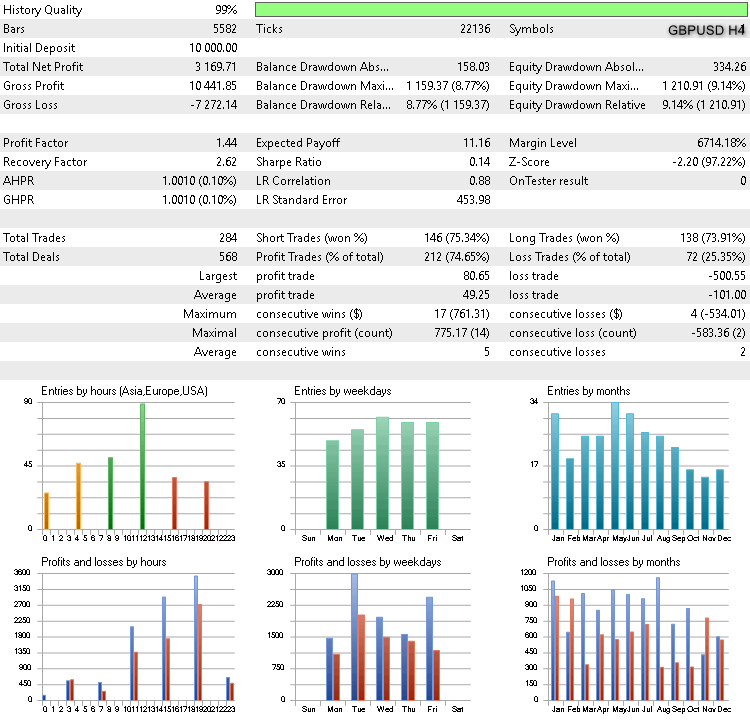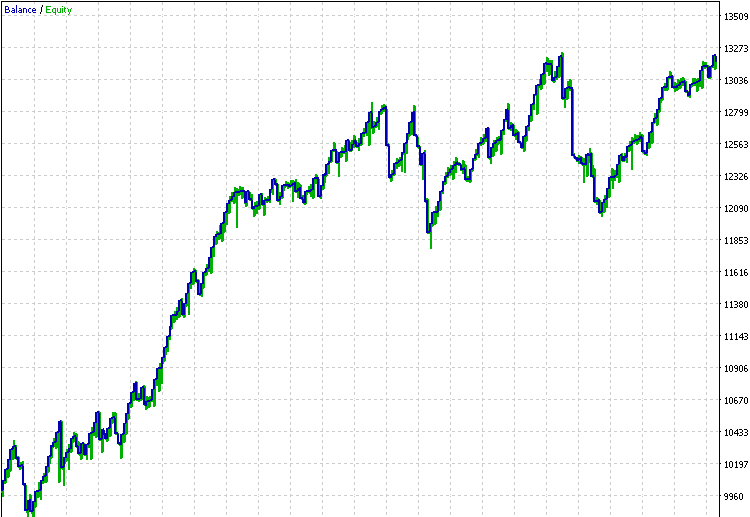JPYUSD H1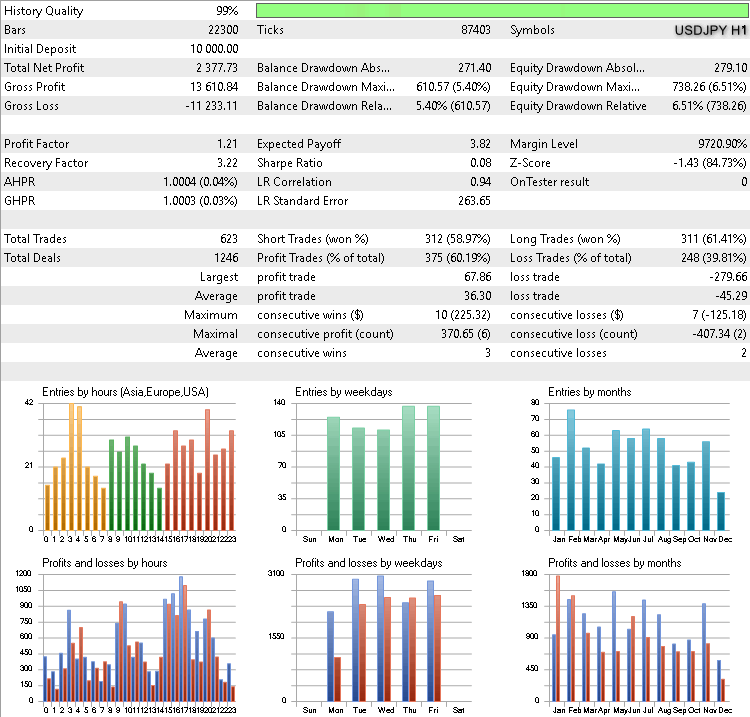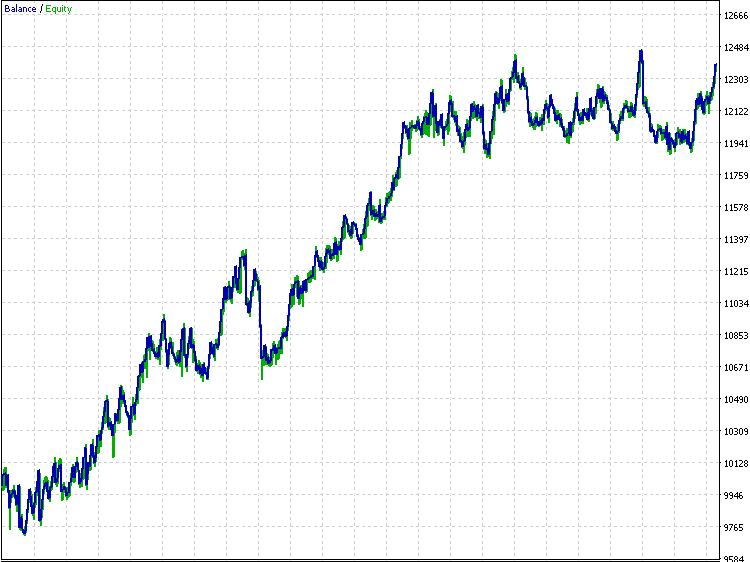JPYUSD H4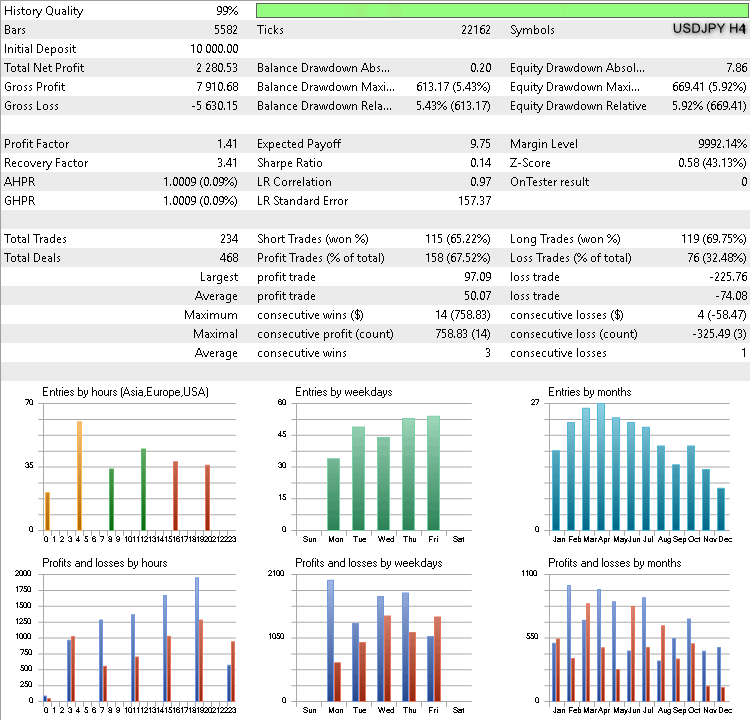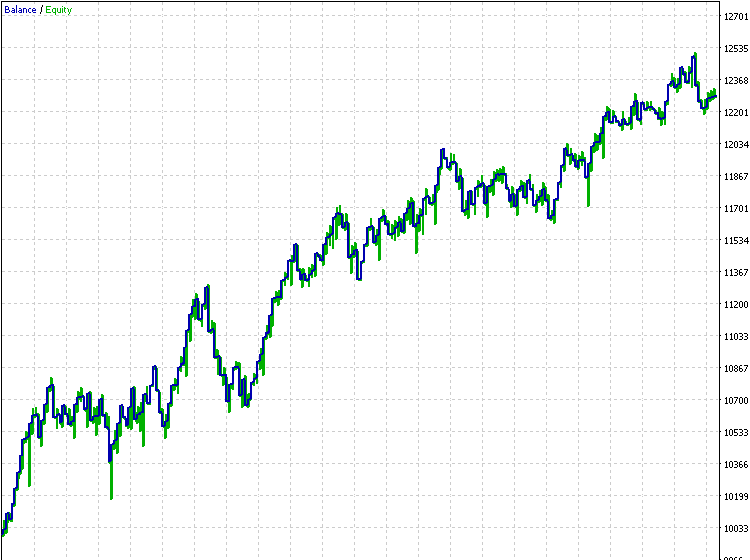### 结束语

#      名称         类型                           说明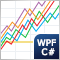优化管理(第二部分)：创建按键对象和附加逻辑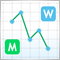美林(Merrill)形态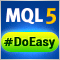轻松快捷开发 MetaTrader 程序的函数库（第十五部分）：品种对象集合轻松快捷开发 MetaTrader 程序的函数库 (第十六部分) : 品种集合事件